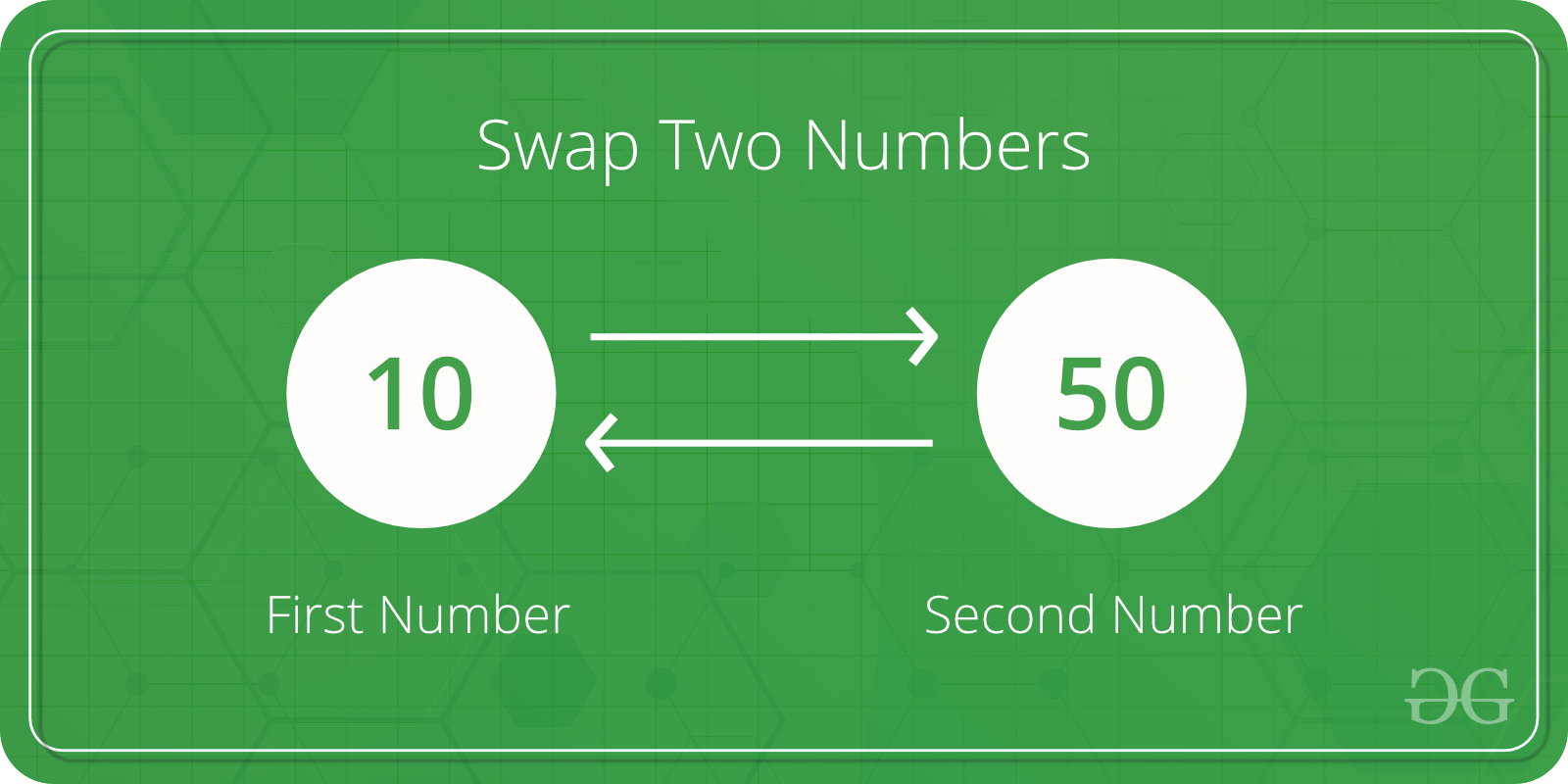Open in App
Not now

# Python Program to Swap Two Variables

• Difficulty Level : Easy
• Last Updated : 08 Jul, 2021

Given two variables x and y, write a Python program to swap their values. Let’s see different methods in Python to do this task.Method 1: Using Naive approach
The most naive approach is to store the value of one variable(say x) in a temporary variable, then assigning the variable x with the value of variable y. Finally, assign the variable y with the value of the temporary variable.

## Python3

 `# Python program to demonstrate``# swapping of two variables` `x ``=` `10``y ``=` `50` `# Swapping of two variables``# Using third variable``temp ``=` `x``x ``=` `y``y ``=` `temp` `print``(``"Value of x:"``, x)``print``(``"Value of y:"``, y)`

Output

```Value of x: 50
Value of y: 10```

Method 2: Using comma operator
Using the comma operator the value of variables can be swapped without using a third variable.

## Python3

 `# Python program to demonstrate``# swapping of two variables`  `x ``=` `10``y ``=` `50` `# Swapping of two variables``# without using third variable``x, y ``=` `y, x` `print``(``"Value of x:"``, x)``print``(``"Value of y:"``, y)`

Output

```Value of x: 50
Value of y: 10```

Method 3: Using XOR
The bitwise XOR operator can be used to swap two variables. The XOR of two numbers x and y returns a number which has all the bits as 1 wherever bits of x and y differ. For example XOR of 10 (In Binary 1010) and 5 (In Binary 0101) is 1111 and XOR of 7 (0111) and 5 (0101) is (0010).

## Python3

 `# Python program to demonstrate``# Swapping of two variables` `x ``=` `10``y ``=` `50` `# Swapping using xor``x ``=` `x ^ y``y ``=` `x ^ y``x ``=` `x ^ y` `print``(``"Value of x:"``, x)``print``(``"Value of y:"``, y)`

Output

```Value of x: 50
Value of y: 10```

Method 4: Using arithmetic operators we can perform swapping in two ways.

• Using addition and subtraction operator :

The idea is to get sum in one of the two given numbers. The numbers can then be swapped using the sum and subtraction from sum.

## Python3

 `# Python program to demonstrate``# swapping of two variables` `x ``=` `10``y ``=` `50` `# Swapping of two variables``# using arithmetic operations``x ``=` `x ``+` `y  ``y ``=` `x ``-` `y ``x ``=` `x ``-` `y` `print``(``"Value of x:"``, x)``print``(``"Value of y:"``, y)`

Output

```Value of x: 50
Value of y: 10```
• Using multiplication and division operator :

The idea is to get multiplication of the two given numbers. The numbers can then be calculated by using the division.

## Python3

 `# Python program to demonstrate``# swapping of two variables` `x ``=` `10``y ``=` `50` `# Swapping of two numbers``# Using multiplication operator` `x ``=` `x ``*` `y``y ``=` `x ``/` `y``x ``=` `x ``/` `y` `print``(``"Value of x : "``, x)``print``(``"Value of y : "``, y)`

Output

```Value of x :  50.0
Value of y :  10.0```

Method 5: using Bitwise addition and subtraction for swapping.

## Python3

 `#Python program to demonstrate``#swapping of two numbers``a ``=` `5``b ``=` `1``a ``=` `(a & b) ``+` `(a | b)``b ``=` `a ``+` `(~b) ``+` `1``a ``=` `a ``+` `(~b) ``+` `1``print``(``"a after swapping: "``, a)``print``(``"b after swapping: "``, b)`

Output

```a after swapping:  1
b after swapping:  5```

My Personal Notes arrow_drop_up